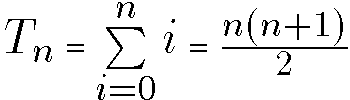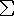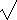# Definitions, Uses, Data Types, and Levels of Measurement

#### Lesson Overview

The term statistics has several basic meanings. First, statistics is a subject or field of study closely related to mathematics. This four week, sixteen lesson unit will first introduce and briefly cover the area known as descriptive statistics.

 Descriptive statistics generally characterizes or describes a set of data elements by graphically displaying the information or describing its central tendancies and how it is distributed.

The last half of the course will cover inferential statistics.

 Inferential statistics tries to infer information about a population by using information gathered by sampling.

 Statistics: The collection of methods used in planning an experiment and analyzing data in order to draw accurate conclusions.

### General Terms Used Throughout Statistics

 Population: The complete set of data elements is termed the population.

The term population will vary widely with its application. Examples could be any of the following proper subsets: animals; primates; human beings; homo sapiens; U.S. citizens; who are attending Andrews University, as graduate students, in the School of Education, as Masters students, female, last name starting with S, who web registered.

 Sample: A sample is a portion of a population selected for further analysis.

How samples are obtained or types of sampling will be studied in lesson 7. Most any of the examples above for population could serve as a sample for the next higher level data set.

 Parameter: A parameter is a characteristic of the whole population.

 Statistic: A statistic is a characteristic of a sample, presumably measurable.

The plural of statistic just above is another basic meaning of statistics.

Assume there are 8 students in a particular statistics class, with 1 student being male. Since 1 is 12.5% of 8, we can say 13% are male. The 13% represents a parameter (not a statistic) of the class because it is based on the entire population. If we assume this class is representative of all classes, and we treat this 1 student as a sample drawn from a larger population, then the 13% becomes a statistic.

 Remember: Parameter is to Population as Statistic is to Sample.

Inferential statistics is used to draw conclusions about a population by studying a sample. It is not guesswork! We test hypotheses about a parameter's value with a certain risk of being wrong. That risk is carefully specified. Also, descriptive and inferential statistics are not mutually exclusive. The inferences made about a population from a sample help describe that population. We also tend to use Roman letters for statistics and Greek letters for parameters.

### Basic Mathematics for Statistics

This course will avoid complex models utilizing complicated mathematics. You will need to be familiar with, however, the fundamental arithmetic operations, elementary algebra, and some basic symbolism.

An interesting subset of the natural numbers generated by addition are called Triangular Numbers. These are so called because these are the total number of dots, if we arrange the dots in a triangle with one additional dot in each layer.

 • • • • • • • • • •
The triangular numbers thus are: 0, 1, 3, 6, 10, 15, 21, ....

Suppose we wish to add together the first 100 natural numbers, which is equivalent to finding the 100th triangular number. One way to do this is by grouping them as follows:

 T100 = (1 +100) + (2+99) + (3 + 98) + ... + (50 + 51) = 101• 50 = 101• 100/2

In general we write:where mathematicians use the capital Greek letter(sigma) to represent summation. Your teacher has a particular fondness for this symbol since the first computer he had much access to had that nickname.

There are three important rules for using the summation operator:

1. Since multiplication distributes over addition, the sum of a constant times a set of numbers is the same as the constant times the sum of the set of numbers.

Example: Cx1 + Cx2 + ... + Cxn = C(x1 + x2 + ...+ xn)

2. The sum of a series of constants is the same as N times the constant, where N represents how many constants there are.

Example: 4 + 4 + 4 + 4 + 4 = 5 × 4 = 20.

3. Since addition is commutative, the total sum of two or more scores for several individuals can be achieved either by summing the scores separately and then combining them or by summing an individual's scores and then combining them.

Example: Joe got scores of 500 and 550 for his verbal and quantitative SAT scores whereas Jim got scores of 520 and 510, respectively. 500 + 550 + 520 + 510 = 1050 + 1030 = 500 + 520 + 550 + 510 = 1020 + 1060 = 2090.

In addition to the operations of addition, subtraction, multiplication, and division, several other arithmetic operators often appear. Exponentiation and absolute value are two such. Also, various symbols of inclusion (parentheses, brackets, braces, vincula) are used.

Exponentiation is a general term which includes squaring (122=144), cubing (63=216), and square roots (16½=(16)=4. When the square root symbol (surd and symbol of inclusion, in recent history a vinculum, but historically parentheses) is used, we general (although not quite always) mean only the positive square root.

The absolute value operator indicates the distance (always non-negative) a number is from the origin (zero). The symbol used is a vertical line on either side of the operand. Thus, if x>0, then |x|=x, if x<0, then |x|=-x, and if x=0, |x|=0.(x2)=|x|.

There is a proscribed order for arithmetic operations to be performed.

Example: If we write 4 × 5 + 3 it is conventional to multiply the 4 and 5 together before adding the 3 and thus obtain 23. Some calculators are algebraic and handle this appropriately, others do not.

Parentheses and other symbols of inclusion are used to modify the normal order of operations. We say these symbols of inclusion have the highest priority or precidence.

Exponentiation is done next. There is confusion when exponents are stacked which we will not deal with here except to say computer scientists tend to do it from left to right while mathematicians know that is wrong.

Multiplication and Division are done next, in order, from left to right.

Addition and Subtraction are done next, in order, from left to right.

A mnemonic such as Please Eat Miss Daisy's Apple Sauce can be useful for remembering the proper order of operation.

### Accuracy vs. Precision

The distinction between accuracy and precision, reviewed in Numbers lesson 9, is very important.

This ties in with significant figures, and proper rounding of results. I have several major concerns regarding significant digits.

1. There needs to be sufficient (not to few). Slide rule accuracy or three significant digits has a long-standing precident in science. We are not doing science here so two may suffice, but rarely one.
2. There should not be too many significant digits. Generally, more than 5 is probably a joke, especially in the "softer" sciences. Thus representing 1/3 or 1/7 with infinite precision (by indicating the repeated unit) should not occur.
3. Care must be taken so that a primary statistics (such as variance) is not incorrectly derived from a secondary statistic (such as standard deviation) in such a way that accuracy is lost. We will discuss this more in textbook Chapter 3.
4. A mean and standard deviation or mean and margin of error should be given to compatible precision.
5. There are proper rules, but they are difficult to explain to the general public. Thus every statistics book gives its own heuristic.

### Uses and Abuses of Statistics

Most of the time, samples are used to infer something (draw conclusions) about the population. If an experiment or study was done cautiously and results were interpreted without bias, then the conclusions would be accurate. However, occasionally the conclusions are inaccurate or inaccurately portrayed for the following reasons:
• Sample is too small.
• Even a large sample may not represent the population.
• Unauthorized personnel are giving wrong information that the public will take as truth. A possibility is a company sponsoring a statistics research to prove that their company is better.
• Visual aids may be correct, but emphasize different aspects. Specific examples include graphs which don't start at zero thus exaggerating small differences and charts which misuse area to represent proportions. Often a chart will use a symbol which is both twice as long and twice as high to represent something twice as much. The area, in this case however, is four times as much!
• Precise statisitics or parameters may incorrectly convey a sense of high accuracy.
• Misleading or unclear percentages are often used.
Statistics are often abused. Many examples could be added, (even books have been written) but it will be more instructive and fun to find them on your own.

### Types of Data

A dictionary defines data as facts or figures from which conclusions may be drawn. Thus, technically, it is a collective, or plural noun. Some recent dictionaries acknowledge popular usage of the word data with a singular verb. However we intend to adhere to the traditional "English" teacher mentality in our grammar usage—sorry if "data are" just doesn't sound quite right! (My mother and step-mother were both English teachers, so clearly no offense is intended above.) Datum is the singular form of the noun data. Data can be classified as either numeric or nonnumeric. Specific terms are used as follows:
1.  Qualitative data are nonnumeric.

{Poor, Fair, Good, Better, Best}, colors (ignoring any physical causes), and types of material {straw, sticks, bricks} are examples of qualitative data.

Qualitative data are often termed catagorical data. Some books use the terms individual and variable to reference the objects and characteristics described by a set of data. They also stress the importance of exact definitions of these variables, including what units they are recorded in. The reason the data were collected is also important.

2.  Quantitative data are numeric.

Quantitative data are further classified as either discrete or continuous.

•  Discrete data are numeric data that have a finite number of possible values.

A classic example of discrete data is a finite subset of the counting numbers, {1,2,3,4,5} perhaps corresponding to {Strongly Disagree... Strongly Agree}.

Another classic is the spin or electric charge of a single electron. Quantum Mechanics, the field of physics which deals with the very small, is much concerned with discrete values.

When data represent counts, they are discrete. An example might be how many students were absent on a given day. Counts are usually considered exact and integer. Consider, however, if three tradies make an absence, then aren't two tardies equal to 0.67 absences?

•  Continuous data have infinite possibilities: 1.4, 1.41, 1.414, 1.4142, 1.141421...
The real numbers are continuous with no gaps or interruptions. Physically measureable quantities of length, volume, time, mass, etc. are generally considered continuous. At the physical level (microscopically), especially for mass, this may not be true, but for normal life situations is a valid assumption.

The structure and nature of data will greatly affect our choice of analysis method. By structure we are referring to the fact that, for example, the data might be pairs of measurements. Consider the legend of Galileo dropping weights from the leaning tower of Pisa. The times for each item would be paired with the mass (and surface area) of the item. Something which Galileo clearly did was measure the time it took a pendulum to swing with various amplitudes. (Galileo Galilei is considered a founder of the experimental method.)

### Levels of Measurement

The experimental (scientific) method depends on physically measuring things. The concept of measurement has been developed in conjunction with the concepts of numbers and units of measurement. Statisticians categorize measurements according to levels. Each level corresponds to how this measurement can be treated mathematically.

1.  Nominal: Nominal data have no order and thus only gives names or labels to various categories.

2.  Ordinal: Ordinal data have order, but the interval between measurements is not meaningful.

3.  Interval: Interval data have meaningful intervals between measurements, but there is no true starting point (zero).

4.  Ratio: Ratio data have the highest level of measurement. Ratios between measurements as well as intervals are meaningful because there is a starting point (zero).

Nominal comes from the Latin root nomen meaning name. Nomenclature, nominative, and nominee are related words. Gender is nominal. (Gender is something you are born with, whereas sex is something you should get a license for.)

Example 1: Colors
To most people, the colors: black, brown, red, orange, yellow, green, blue, violet, gray, and white are just names of colors.

To an electronics student familiar with color-coded resistors, this data is in ascending order and thus represents at least ordinal data.

To a physicist, the colors: red, orange, yellow, green, blue, and violet correspond to specific wavelengths of light and would be an example of ratio data.

Example 2: Temperatures
What level of measurement a temperature is depends on which temperature scale is used.
Specific values: 0°C = 32°F = 273.15 K = 491.69°R     100°C = 212°F = 373.15 K = 671.67°R     -17.8°C = 0°F = 255.4 K = 459.67°R
where C refers to Celsius (or Centigrade before 1948); F refers to Fahrenheit; K refers to Kelvin; R refers to Rankine.

Only Kelvin and Rankine have true zeroes (starting point) and ratios can be found. Celsius and Fahrenheit are interval data; certainly order is important and intervals are meaningful. However, a 180° dashboard is not twice as hot as the 90° outside temperature (Fahrenheit assumed)! Rankine has the same size degree as Fahrenheit but is rarely used. To interconvert Fahrenheit and Celsius, see Numbers lesson 12. (Note that since 1967, the use of the degree symbol on tempertures Kelvin is no longer proper.)

Although ordinal data should not be used for calculations, it is not uncommon to find averages formed from data collected which represented Strongly Disagree, ..., Strongly Agree! Also, averages of nominal data (zip codes, social security numbers) is rather meaningless!